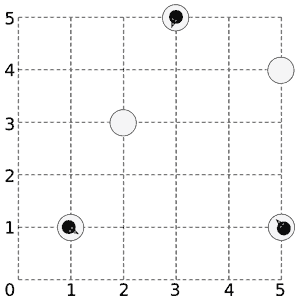March of the Penguins

Description

Somewhere near the south pole, a number of penguins are standing on a number of ice floes. Being social animals, the penguins would like to get together, all on the same floe. The penguins do not want to get wet, so they have use their limited jump distance to get together by jumping from piece to piece. However, temperatures have been high lately, and the floes are showing cracks, and they get damaged further by the force needed to jump to another floe. Fortunately the penguins are real experts on cracking ice floes, and know exactly how many times a penguin can jump off each floe before it disintegrates and disappears. Landing on an ice floe does not damage it. You have to help the penguins find all floes where they can meet.A sample layout of ice floes with 3 penguins on them.

Input

On the first line one positive number: the number of testcases, at most 100. After that per testcase:

• One line with the integer N (1 ≤ N ≤ 100) and a floating-point number D (0 ≤ D100 000), denoting the number of ice pieces and the maximum distance a penguin can jump.

• N lines, each line containing xi, yi, ni and mi, denoting for each ice piece its X and Y coordinate, the number of penguins on it and the maximum number of times a penguin can jump off this piece before it disappears (−10 000xi, yi10 000, 0 ≤ ni ≤ 10, 1 ≤ mi ≤ 200).

Output

Per testcase:

• One line containing a space-separated list of 0-based indices of the pieces on which all penguins can meet. If no such piece exists, output a line with the single number −1.

Sample Input

`25 3.51 1 1 12 3 0 13 5 1 15 1 1 15 4 0 13 1.1-1 0 5 100 0 3 92 0 1 1`

Sample Output

`1 2 4-1`

#include <stdio.h>
#include
<iostream>
#include
<cmath>
#include
<string.h>
using namespace std;
const int N=20010;
const int MAXE=200010;
const int inf = 1<<29;
struct Edge
{

int v, next, w;
}edge[MAXE
*8];
int head[N], pre[N], cur[N], level[N], gap[N];
int cnt;
void addedge(int u, int v, int w)
{
edge[cnt].v
= v;
edge[cnt].w
= w;
edge[cnt].next
= cnt++;
edge[cnt].v
= u;
edge[cnt].w
= 0;
edge[cnt].next
= cnt++;
}
int sap(int s, int t, int n)
{

int flow = 0, aug = inf;

int u;

bool flag;

for(int i = 0; i <= n; i++)
{
cur[i]
gap[i]
= level[i] = 0;
}
gap[s]
= n;
u
= pre[s] = s;

while (level[s] < n)
{
flag
= 0;

for (int &= cur[u]; j != -1; j = edge[j].next)
{

int v = edge[j].v;

if (edge[j].w > 0 && level[u] == level[v] + 1)
{
flag
= 1;

if (edge[j].w < aug) aug = edge[j].w;
pre[v]
= u;
u
= v;

if (u == t)
{
flow
+= aug;

while (u != s)
{
u
= pre[u];
edge[cur[u]].w
-= aug;
edge[cur[u]
^1].w += aug;
}
aug
= inf;
}

break;
}
}

if(flag)

continue;

int mindis = n;

for(int j = head[u]; j != -1; j = edge[j].next)
{

int v = edge[j].v;

if(edge[j].w > 0 && level[v] < mindis)
{
mindis
= level[v];
cur[u]
= j;
}
}

if((--gap[level[u]]) == 0)

break;
gap[level[u]
=mindis+1]++;
u
= pre[u];
}

return flow;
}
struct P
{

int x, y, cap, time;
}floes[
110];
int redis(P& a, P& b)
{

return (a.x - b.x) * (a.x - b.x) + (a.y - b.y) * (a.y - b.y);
}
void build (int s, int t, int ver, int n, double maxdis)
{

int i, j;
cnt
= 0;

for (i = 0; i <= ver; i++)
{
= -1;
}

for (i = 1; i <= n; i++)
{
+ n, floes[i].time);
}

for (i = 1; i <= n; i++)
{

for (j = 1; j <= n; j++)
{

if (maxdis * maxdis > (double)redis(floes[i], floes[j]))
{
+ n, j, inf);
}
}
}
}
int main()
{

int ca, n, sum, i, s, t, ver;

double maxdis;
scanf(
"%d"&ca);

while (ca--)
{
scanf(
"%d%lf"&n, &maxdis);

int s = 0, t, ver = n + n + 1;

for (i = 1, sum = 0; i <= n; i++)
{
scanf(
"%d%d%d%d"&floes[i].x, &floes[i].y, &floes[i].cap, &floes[i].time);
sum
+= floes[i].cap;
}

int sign;

for (i = 1, sign = 0; i <= n; i++)
{
t
= i;
build (s, t, ver, n, maxdis);

int ans = sap(s, t, ver);

if (ans == sum)
{
sign
= 1;
printf(
"%d ", t - 1);
}
}

if (!sign) printf("-1");
printf(
"\n");

}

return 0;
}